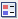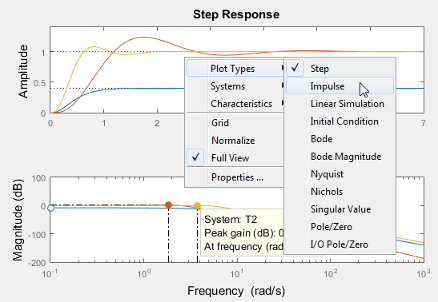Main Content

## Joint Time-Domain and Frequency-Domain Analysis

This example shows how to compare multiple types of responses side by side, including both time-domain and frequency-domain responses, using the interactive Linear System Analyzer app.

Obtain models whose responses you want to compare.

For example, compare a third-order plant `G`, and the closed-loop responses of `G` with two different controllers, `C1` and `C2`.

```G = zpk([],[-5 -5 -10],100); C1 = pid(0,4.4); T1 = feedback(G*C1,1); C2 = pid(2.9,7.1); T2 = feedback(G*C2,1); ```

Open the Linear System Analyzer tool to examine the responses of the plant and the closed-loop systems.

`linearSystemAnalyzer(G,T1,T2)`By default, the Linear System Analyzer launches with a plot of the step response of the three systems. Clickto add a legend to the plot.

Add plots of the impulse responses to the Linear System Analyzer display.

In the Linear System Analyzer, select Edit > Plot Configurations to open the Plot Configurations dialog box.Select the two-plot configuration. In the Response Type area, select `Bode Magnitude` for the second plot type.Click to add the Bode plots to the Linear System Analyzer display.

Display the peak values of the Bode responses on the plot.

Right-click anywhere in the Bode Magnitude plot and select Characteristics > Peak Response from the menu.Markers appear on the plot indicating the peak response values. Horizontal and vertical dotted lines indicate the frequency and amplitude of those responses. Click on a marker to view the value of the peak response in a datatip.You can use a similar procedure to select other characteristics such as settling time and rise time from the Characteristics menu and view the values.

You can also change the type of plot displayed in the Linear System Analyzer. For example, to change the first plot type to a plot of the impulse response, right-click anywhere in the plot. Select Plot Types > ImpulseThe displayed plot changes to show the impulse of the three systems.

## Support

#### Learn how to automatically tune PID controller gains

Download code examples